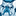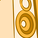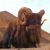# QlikView Documents

QlikView documentation and resources.

Announcements
Customer & Partners, DEC. 9, 11 AM ET: Qlik Product & Strategy Roadmap Session: Data Analytics REGISTER NOW

# Remove Empty columns (All Null values) in script dynamicallyMVP

## Remove Empty columns (All Null values) in script dynamically

Hi All,

The below script helps to remove the empty columns (which has all null values) dynamically from the DataModel.  To use just copy the below script and paste at the end of your script.

```FOR a = 0 TO NoOfTables() - 1
LET vTable = TableName(\$(a));
LET d = 0;
FOR i = 1 TO NoOfFields('\$(vTable)')
LET j = i - d;
LET vField = FieldName(\$(j), '\$(vTable)');
LET vFieldValueCount = Alt(FieldValueCount('\$(vField)'), 0);
IF (vFieldValueCount <= 0 ) THEN
DROP FIELD [\$(vField)] FROM \$(vTable);
TRACE DROP FIELD [\$(vField)] FROM \$(vTable);
LET d = d + 1; // dropping fields impacts the internal field no.
END IF
NEXT i
NEXT a
```

If you want the empty values (whitespaces) then use below script

```FOR a = 0 TO NoOfTables() - 1
LET vTable = TableName(\$(a));
LET d = 0;
FOR i = 1 TO NoOfFields('\$(vTable)')
LET j = i - d;
LET vField = FieldName(\$(j), '\$(vTable)');
DropField:
Resident \$(vTable)
WHERE Len(Trim(\$(vField))) > 0;

LET vNoOfRows = NoOfRows('DropField');
DROP TABLE  DropField;
IF vNoOfRows = 0  THEN
DROP FIELD [\$(vField)] FROM \$(vTable);
TRACE DROP FIELD [\$(vField)] FROM \$(vTable);
LET d = d + 1; // dropping fields impacts the internal field no.
END IF
NEXT i
NEXT a
```

You can test the above script using below Inline table.

Data:

*,

' ' AS Field3,

Null() AS Field4

INLINE [

Field1, Field2

1,2

3,4

5,6];

When you use the first scrip then Field4 will be dropped, and when you use second script then both Field3 and Field4 are dropped.

Hope this helps.

Regards,

jagan.

Labels (3)

• ### ScriptingMaster II

Excellent code, Jagan. This is very helpfulContributor III

Hi,

do you have also a solution for deleting columns which have e.g. only 10% values? E.g. you have 5 fields which have 100 values and 1 field which have only 10 values....Not applicableMaster II

It would be great to send the [\$(vField)] to a .txt file

So Fields with Empty Data could be sent to a emptyfields.txt file.

I tried to use STORE, but they are not tables, so.....

Any ideas?Partner

FROM \$(vTable)

should be

FROM [\$(vTable)]

To deal with spaces in table names...Partner
2.     Dropped field, from Table
3.     dummy, dummy
4. ];
5. FOR a = 0 TO NoOfTables() - 1
6.     LET vTable = TableName(\$(a));
7.     LET d = 0
8.     FOR i = 1 TO NoOfFields('\$(vTable)'
9.         LET j = i - d;
10.         LET vField = FieldName(\$(j), '\$(vTable)');
11.         LET vFieldValueCount = Alt(FieldValueCount('\$(vField)'), 0);
12.         IF (vFieldValueCount <= 0 ) THEN
13.          Concatenate(Dropped) LOAD * INLINE [
14.          Dropped field, from Table
15.               "\$(vField)", "\$(vTable)"
16.                  ];
17.          DROP FIELD [\$(vField)] FROM [\$(vTable)];
18.          TRACE DROP FIELD [\$(vField)] FROM [\$(vTable)];
19.          LET d = d + 1; // dropping fields impacts the internal field no.
20.         END IF
21.        NEXT i
22.     NEXT a
1. Store Dropped into Dropped.csv (csv);Specialist

It would be great to send the [\$(vField)] to a .txt file?

Try this:

Catch_dropped_fields:

Concatenate(Catch_dropped_fields) LOAD '\$(vField)' as [dropped field] AutoGenerate(1);

The total statement would be:

Catch_dropped_fields:

FOR a = 0 TO NoOfTables() - 1

LET vTable = TableName(\$(a));

LET d = 0;

FOR i = 1 TO NoOfFields('\$(vTable)')

LET j = i - d;

LET vField = FieldName(\$(j), '\$(vTable)');

LET vFieldValueCount = Alt(FieldValueCount('\$(vField)'), 0);

IF (vFieldValueCount <= 0 ) THEN

Concatenate(Catch_dropped_fields) LOAD '\$(vField)' as [dropped field] AutoGenerate(1);

DROP FIELD [\$(vField)] FROM [\$(vTable)];

TRACE DROP FIELD [\$(vField)] FROM \$(vTable);

LET d = d + 1; // dropping fields impacts the internal field no.

END IF

NEXT i

NEXT aContributor II

Hi, Jagan this code really help us

Can you please let me know How to load all tables and all fields  dynamically from local directory if those are not empty

Version history
Last update:
‎2015-04-07 05:39 AM
Updated by: